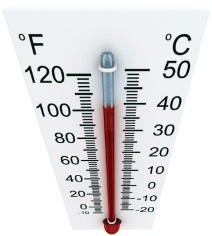Celsius and Fahrenheit belongs to two different scales of temperature, with Fahrenheit usually used in the US while Celsius, which belongs to the metric scale, is used worldwide. They use different temperature readings for what may be the same reference point. For example, at normal pressure, boiling water is measured at a temperature of 100° in Celsius, but 212° in Fahrenheit. It's not difficult to convert Fahrenheit to Celsius or to convert Celsius to Fahrenheit. All you need to do is to use simple addition, subtraction, multiplication, and division. Below is the clear instruction as well as several examples to help you better solve this problem.## How to Convert Celsius to Fahrenheit

### Method 1: Use 9/5

The Formula: °F = (°C × 9/5) + 32

How to Do:

Step 1: Take the temperature in Celsius and multiply that number by 9 and then devide by 5, or if you are good at fraction, just mulitiply the temperature in Celsius by 9/5

Step 2: Add 32 degrees to that number

Step 3: The result you get is degrees Fahrenheit.

### Method 2: Use 1.8 instead of 9/5

The Formula: °F = °C × 1.8 + 32

How to Do:

Step 1: Take the temperature in Celsius and multiply by 1.8

Step 2: Add 32 degrees to that number

Step 3: The result you get is degrees Fahrenheit.

### Example: Convert 21°C (room temperature) to Fahrenheit

Step 1: multiply 21 with 9/5 or 1.8 and the result is 37.8

Step 2: Add 32 degrees to that number

Step 3: The result you get is degrees Fahrenheit: 69.8°F

## How to Convert Fahrenheit to Celsius

### Method 1: multiply by 5/9

The Formula: °C = (°F - 32) × 5/9

How to Do:

Step 1: Take the temperature in Fahrenheit and subtract 32 from it

Step 2: multiply that number by 5/9 (or multiply it by 5 and then divide by 9)

Step 3: The result you get is degrees Celsius.

Example: Convert 50°F (a cool day) to Celsius

Step 1: Subtract 32 from 50 and the result is 18

Step 2: Multiply 18 by 5/9

Step 3: The result you get is degrees Celcius: 10°C

### Method 2: divide by 1.8

The Formula: °C = (°F - 32) ÷ 1.8

How to Do:

Step 1: Take the temperature in Fahrenheit and subtract 32 from it

Step 2: Divide that number by 1.8

Step 3: The result you get is degrees Celsius.

Example: Convert 50°F (a cool day) to Celsius

Step 1: Subtract 32 from 50 and the result is 18

Step 2: Divide 18 by 1.8

Step 3: The result you get is degrees Celcius: 10°C

Notes: for your convenience, you can always use an online caculator to get an accuate conversion between celcius and Fahrenheit, check the link below:

http://www.onlineconversion.com/temperature.htm

## Examples: Temperatures in Both Celsius and Fahrenheit

After knowing how to convert Celsius to Fahrenheit, below are some examples to help you get a clearer idea:
 Celsius (°C) Fahrenheit (°F) 100°C ( water boiling point) 212°F ( water boiling point) 37°C ( normal body temperature) 98.6°F ( normal body temperature) 0°C ( water freezing point) 32°F ( water freezing point) -10°C 14 °F 5°C 41°F 10°C 50°F 15°C 59°F 20°C 68°F 25°C 77°F 30°C 86°F 35°C 95°F 40°C 104°F

The following video derives the formulas used to convert temperature between Celsius and Fahrenheit and the provided conversion examples: